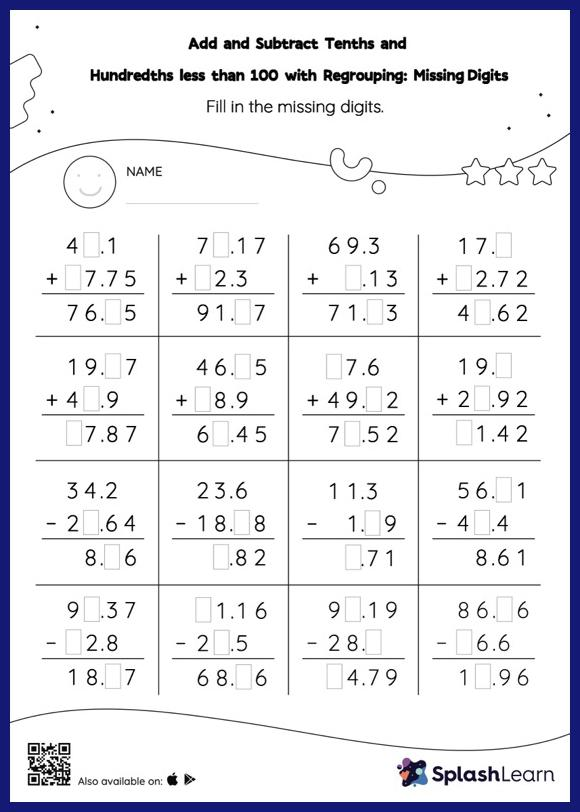# Add and Subtract Tenths and Hundredths less than 100 with Regrouping: Missing Digits Worksheet

Home > Add and Subtract Tenths and Hundredths less than 100 with Regrouping: Missing DigitsAdd and subtract tenths and hundredths less than 100 with regrouping worksheet is a great way to help learners become fluent with the concept of addition and subtraction. Students use the relationship between addition and subtraction to find the missing number while adding or subtracting decimals. To reach the answer, they must also regroup the numbers in add and subtract tenths and hundredths less than 100 with regrouping worksheet using the relationships between ones, tenths, hundredths, thousandths, etc.